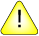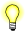This version of this document is no longer maintained. For the latest documentation, see http://www.qnx.com/developers/docs.

# bc

"Bench calculator" arbitrary-precision arithmetic language (POSIX)

## Syntax:

`bc [-l] [file...]`

Neutrino

## Options:

-l
("el") Include a library of defined math functions and set the scale to 20. (See "Library functions," below.)
file
The pathname of a text file containing commands and function definitions to be interpreted by bc. If any files are specified, bc processes those files, then reads from the standard input.

## Description:

The bc utility is an interactive, programmable calculator that supports a complete set of control structures, including functions.

The bc utility supports 26 functions, 26 simple variables, and 26 array variables. Each array may have up to 2048 elements. In addition, the utility performs arithmetic operations using a radix 100 number system with user-definable precision. When you specify the precision, the numbers are exact to that precision, unlike binary floating-point representation where rounding errors may compromise accuracy. The utility also operates in different bases, so you can easily convert numbers from one base to another.

Many common programming language constructs are supported, including:

• if, while, and for statements
• user-defined functions with parameters
• local variables.

The bc utility provides no support for character or string manipulation. The syntax of bc is derived from C, but doesn't constitute a programming language.

Let's look at a very simple bc program:

`"hello, world\n"`

When this program is run, the statement "hello, world" is echoed with a newline character. In general, the result of any expression that isn't assigned to a variable or used in a control structure is echoed. For example, the following statement:

`5^2`

causes bc to respond with 25.

Note that the caret (^) is an integer exponentiation operator: a^b is a raised to the bth power, where b is truncated to an integer in the range -2^31 to +2^31. All of the "usual" arithmetic operators (+, -,*,/,%) are available, but % is the remainder, not the modulus.

### Bases

Two special builtin variables let you choose the base in which numbers are input and output:

ibase
Input base.
obase
Output base.

When numbers are recognized, the input base determines the significance of each digit. For example, the value 66 in base 10 is:

```    6 * 10^0 =  6
+   6 * 10^1 = 60
--
66```

whereas in base 8, it's:

```    6 * 8^0  =  6
+   6 * 8^1  = 48
--
54```

Note that hex numbers are recognized in the different bases and that their values also change with the base. For example, the number FF is valid in base 10 and has the decimal value 165:

```    F(=15) * 10^0 =  15
+   F(=15) * 10^1 = 150
---
165```

Setting the output base results in one of two numerical formats:

• For bases in the range 2 to 16, the format consists of the alphabet 0-9,A-F.
• For bases in the range 17 to 10000, the format consists of a series of decimal digits with groups separated by spaces. In this format, each group represents a digit of significance, thus the number 61 in obase=20 is printed as:
`3 1`

which should be interpreted as:

```    1 * 20^0 =  1
+   3 * 20^1 = 60
--
61```

### Variables

The bc utility supports 26 simple variables, named a through z (case is significant), and 26 arrays, also named a through z. The context determines whether the simple variable or the array is selected. All variables are auto-initialized to 0 and are always available (i.e. there's no declaration statement).

Functions may create local variables (with the auto statement) that hide the global variables of the same name while the function is executing. Arrays are limited to 2048 elements. Variables may be referenced (right-valued) or assigned to (left-valued).

### Assignment operators

The assignment operator has many variations, as in the C language. For example:

`a += x`

means the same as:

`a = a + x`

All of the binary operators have a corresponding assignment operator.

An assignment expression (e.g. a=10) has a right value that's the new value of a. Thus:

`a = b = 10`

assigns 10 to b, then assigns b to a.

The increment and decrement operators may be applied only to variables. The statement:

`b = a++`

is semantically the same as:

`b = a; a = a + 1;`

and the statement:

`b = ++a`

is semantically the same as:

`a = a + 1; b = a;`

The following table summarizes operator precedence and associativity:

Operator Associativity Class
++, -- Nonassociative (increment, decrement)
- Right to left (unary minus)
^ Right to left (exponentiation)
*, /, % Left to right (multiplicative)
+, - Left to right (additive)
==, <=, >=, !=, <, > Left to right (comparative)
= +=, -=, *=, /=, %=, ^=, Right to left (assignment)

### The if statement

The if statement takes the following form:

`if (expr) statement`

The statement is executed only if expr evaluates to a nonzero quantity. Regardless of the value of expr, the next statement is executed.

```if (i > 2047) {
"i exceeds array limit"
i = 2047;         /* limit index */
}
a[i]      /* always print value */```

### Iteration statements

The bc utility supports two iteration statements: while and for. In both of these structures, break means "exit the loop immediately," and continue means "pretend you have finished executing statement."

The while statement has the general form:

`while (expr) statement`

and is executed following this algorithm:

1. If expr is false, go to 4.
2. Execute statement.
3. Go to 1.
4. Proceed.

In the while statement, continue and break are analogous to "Go to 1" and "Go to 4," respectively.

The for statement has the general form:

`for (expr1;expr2;expr3) statement`

and is executed following this algorithm:

1. expr1
2. If expr2 is false, go to 6.
3. Execute statement.
4. Execute expr3.
5. Go to 2.
6. Proceed.

In the for statement, continue and break are analogous to "Go to 4" and "Go to 6," respectively.

### User-defined functions

In bc, you can define named functions that support parameters, local variables, and recursion. A function name is a single lowercase character (a to z). A function has the general form:

`define name(parameter-list) { function-body }`

You can't remove or replace functions; it's an error to attempt to do so. Functions can call other functions, including themselves.

Functions can accept an arbitrary number of parameters, which may be either simple or array variables.Parameters are passed by value, that is, the function is given a private copy of all parameters that remains in effect until the function returns. Notice that arrays are also passed by value; this differs from the C calling convention.

The first statement in a function can be an auto statement, which creates "local" variables for the function. The auto statement has this general form:

auto [variable|array]...

The local variables are used in place of the global variables of the same name until the function returns; the local variables are also initialized to zero (see the example below).

Functions can contain a return statement. The return statement causes the function to exit, discarding any local variables and parameters. The statement may be in either of two forms:

return

Or

return [retval]

where retval is a constant or a variable name. If no specific return statement is given, or if retval is omitted, a value of 0 is returned.

The following example shows various constructs being used in functions:

```/* function s(b[], n)
* return the sum of the first n elements of b[]
*/
define s(b[],n) {
auto t,i;     /* note that the ';'s are for style,
and aren't required. */

if (n > 2048) {
"Array length out of bounds...\n"
return 0;
}
for (i=0; i < n; i++) {
t += b[i];
}
return t;
}```

The function s() is introduced by the define statement, which also reveals the parameter types. It's important that all functions be called with the appropriate types and number of parameters. Failure to do so results in unpredictable errors.All parameters in bc are passed by value, including arrays. This is unlike C, where arrays are always passed by reference.

The auto statement introduces the locally scoped variables t and i. You should use automatic variables to prevent unwanted side effects of using global variables.

### Builtin variables and functions

Several builtin control variables affect how bc operates. The following variables may be set or queried:

Name Function Range Default
scale Minimum precision maintained in calculations 0..100 0
ibase Radix for input 2..10000 numbers 10
obase Radix for output 2..10000 numbers 10

The bc utility provides the following builtin functions:

Function Returns
sqrt(expression) Square root of the value
length(expression) Number of significant decimal digits in the expression
scale(expression) Number of decimal digits to the right of the decimal marker

### Library functions

The bc library is enabled if you invoke bc with the -l option, or if you include the interpretation file /usr/lib/bclib.b in the command line. The library sets the scale to 20, which you can override by assigning a new value. The library defines the following functions:

Syntax Function
e(expression) Exponential function
k(expr1,expr2) Bessel function of integer order
l(expression) Natural logarithm

## Files:

/usr/lib/bclib.b
Contains the bc library, a set of function definitions that are loaded automatically when the -l option is specified. This file must be present for the -l option to work.

## Exit status:

0
Successful completion.
> 0
An error occurred.

## Caveats:

The bc utility's syntax contains a few ambiguities:

• The notion of a statement in bc is either a structured statement or an expression followed by a newline or a semicolon (;). An expression may be nil, thus a single newline forms a statement. This can lead to unexpected behavior, as in the following example, where the while statement never terminates:
```a=0
while (a < 100)
a++
a```

Although the intent is obvious, the newline at the end of the while statement comprises the body of the loop, thus a is never incremented. The C equivalent of this example is:

```a=0;
while (a < 100);```

The following are "correct" versions:

```a=0
while (a < 100) a++```

Or

`while (++a < 100)`

Or

```while (a < 100) {
a++
}```
• The quit statement causes bc to exit when it encounters the statement, not when it attempts to execute it. Thus, the following causes bc to exit immediately:
```if (0) {
quit
}```

and is equivalent to:

`quit`